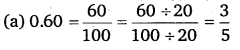# Class 6 Maths NCERT Solutions for Chapter 8 Decimals Ex – 8.2

## Decimals

Question 1.
Complete the table with the help of these boxes and use decimals to write the number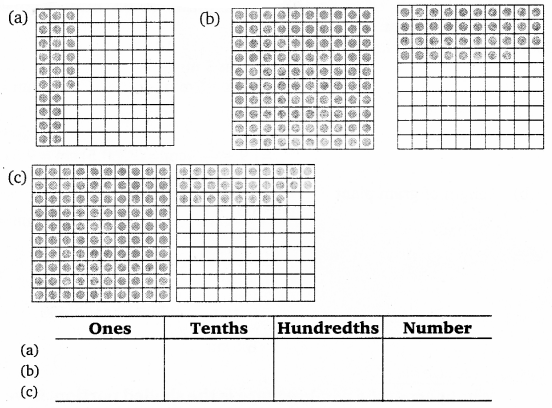Solution:
Completing the table, we have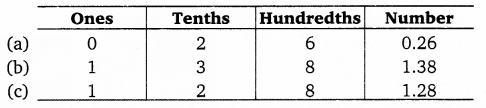Question 2.
Write the numbers given in the following place value table in decimal form: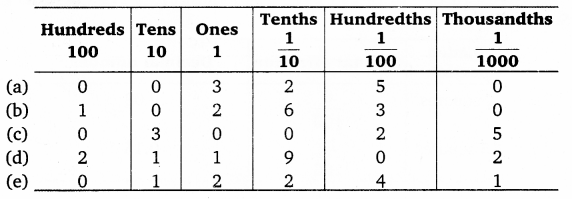Solution: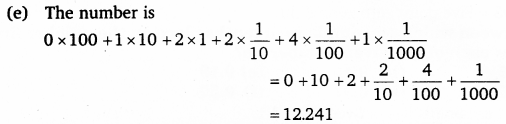Question 3.
Write the following decimals in the place value table:

(a) 0.29
(b) 2.08
(c) 19.60
(d) 148.32
(e) 200.812

Solution:
Let us make a common place value table, assigning appropriate place value to the digits in the given numbers. We have,Question 4.
Write each of the following as decimals: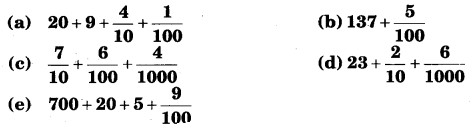Solution: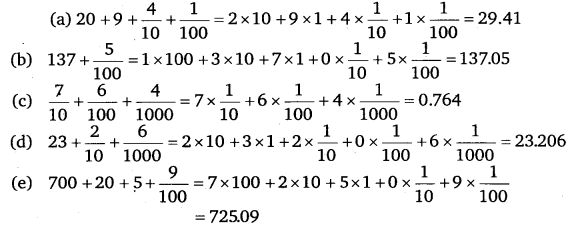Question 5.
Write each of the following decimals in words:

(a) 0. 03
(b) 1.20
(c) 108.56
(d) 10.07
(e) 0. 032
(f) 5.008

Solution:
(a) 0.03 = Zero point zero three or three hundredths.
(b) 1.20 = One point two zero.
(c) 108.56 = One hundred eight point five six.
(d) 10.7 = Ten point zero seven.
(e) 0. 032 = Zero point zero three two.
(f) 5.008 = Five point zero zero eight.

Question 6.
Between which two numbers in tenths place on the number line does each of the given number lie?

(a) 0. 06
(b) 0.45
(c) 0.19
(d) 0.66
(e) 0.92
(f) 0.57

Solution:
All these points lies between 0 and 1.Question 7.
Write as fractions in lowest terms:

(a) 0.60
(b) 0.05
(c) 0.75
(d) 0.25
(f) 0.125
(g) 0.066

Solution: